# Distance Time Graph

## Distance Time Graph - Definition with Examples

Information about motion can be categorized and presented in a number of ways. The data for the total distance travelled in different intervals of time during any translatory motion can be recorded in a table for example.

 Distance Travelled in metres Time Taken in seconds 0 0 2 30 4 60 6 90 8 120

The most popular method for presenting information about distance and time is via a line graph. This kind of line graph presenting distance versus time is popularly known as the distance - time graph. Distance is usually plotted along the y-axis, or the vertical axis while time is usually plotted along the x-axis, or the horizontal axis. Distance - time graph is nothing but a simple line graph that denotes distance versus time findings on the graph.

Drawing such a distance - time line graph is quite simple. First, we must have a set of data about the distances covered and time of an object in motion. Then the axes are drawn with the point of intersection is labelled as O, which stands for the origin. Then, on the two axes we mark the distance and time adhering to a scale which is fixed. Then plot the points by choosing distances against the respective time instants. Join the points with a smooth curve and a distance - time graph is obtained.

Example:

For the following data, the graph is drawn like this

 Distance in metre Time in seconds 0 0 5 10 10 20 15 30 20 40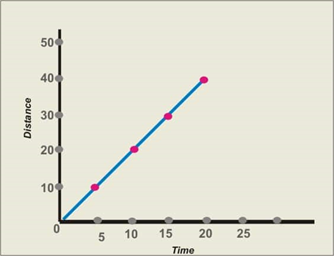By looking at the distance - time graph we will know about the motion of the object. All motion represented on a distance - time graph is unidirectional, since direction cannot be represented by a distance - time graph. If the line is a continuous straight line, then the object has a uniform speed. If the distance remains constant over time, represented by a straight line parallel to the x-axis, then the object is at rest at the given distance. If the graph has curves or lines which are not patternable, then the object is moving with non-uniform speed.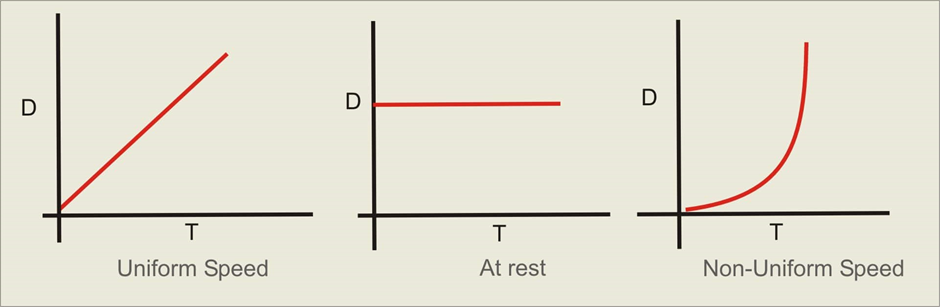Also, if there is a curve in the line drawn on the line graph, it denotes acceleration or deceleration. A curve that is bulged to the right represents acceleration while a curve bulged to the left represents deceleration.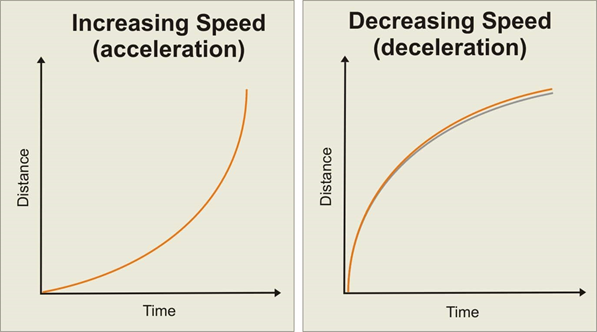Calculating Speed from a distance - time graph:

The speed of an object is the distance travelled by it in unit time. It is possible to calculate the speed of an object, both average and instantaneous using a distance - time graph. The speed of an object is calculated from a distance - time graph as the slope. The slope of a line represents how steep it is. In distance - time graphs, slope, which is the average speed is calculated by dividing a change in y value by the corresponding change in x-value. For the speed at a point of time, if the speed is uniform and the line is straight the speed at that point of time can be calculated by dividing y-value by the corresponding x-value. In case the line is curved due to acceleration or deceleration, that is constantly changing speeds, the instantaneous speed at that point of time can be determined by drawing a tangent at that curve and measuring the slope of that tangent.

Problems:

Question: This diagram shows the motion of a man as he left his house to wander around before coming back. Briefly explain his journey.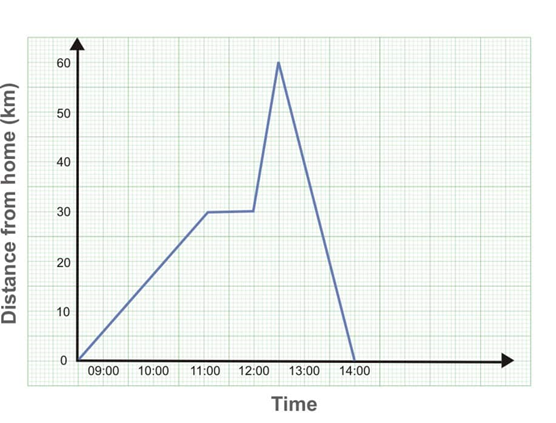Solution: This man started out at 9:00 from his home. He moved at a uniform speed of 15 km/hour for 2 hours and reached 30 km away from his house at 11:00. He was stationary at this distance for one hour. Then he started walking at the speed of 60 km/hour and maintained this speed for half an hour. He then reached the farthest point from home, 60 km away from his home at 12:30. Then he started walking back home, at the speed of 40 km/hour. He finally reached home at 14:00
He walked a 60 km and back, for a total of 120 km. He took 5 hours for this journey and thus reached an average speed of 24 km/hour.

2. From the following graph, find the maximum speed reached by the runner while participating a 1500 metres race.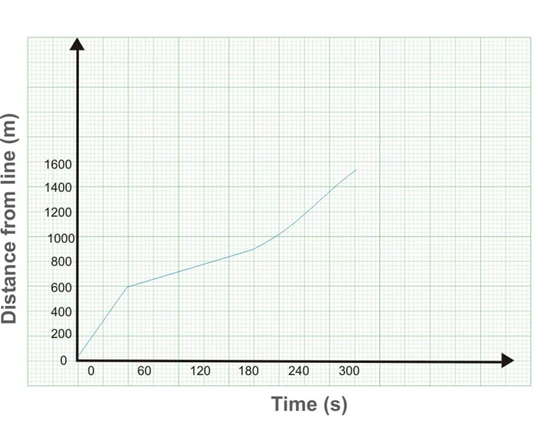We know that the speed can be estimated by looking at the slope on the line of graph in a distance - time graph. As we can clearly see, the slope of the line in the first 600 metres is the highest.

We can assume the speed of the runner was the highest when he hit the 600-metre mark. That is at about 80 seconds. Hence the speed of the runner at that time is 600/80 = 7.5 m/s.

1. This diagram maps a boy’s movements as he left his house to wander around before coming back. Briefly explain his journey.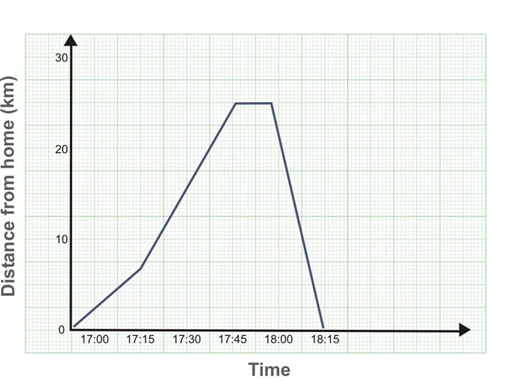This boy left home at 17:00 and advanced 5 km in 15 minutes, at 20 km/hour. He then proceeded to walk at a uniform speed of 40 km/hour, for half an hour, from 17:15 to 17:45. He then stayed at the spot 25 km away from his home for 7.5 minutes. Afterwards, he walked home at the pace of 66.66 km/hour and walked for a total of 22.5 minutes, and finally reached home at 18:45.

The problem with distance - time graph is that it cannot be used to represent velocity or direction. Also, it is difficult o calculate both tiny and large values in the same graph. The simplicity of the graph leads to people taking it for granted and thus misinterpreting data. This also happens when users are not highly familiar with the different parameters for the graphs.